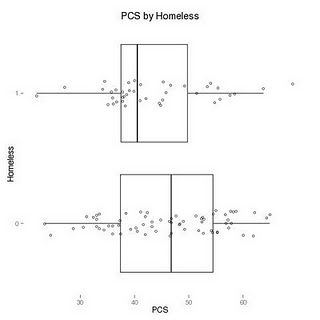Want to share your content on R-bloggers? click here if you have a blog, or here if you don't.Kudos to several of our readers, who suggested simpler ways to craft the graphical display (combination dotplot/boxplot) from our most recent example.

Yihui Xie combines a boxplot with a coarsened version of the PCS scores (using the round() function) used in the stripchart() function.
```ds = read.csv("http://www.math.smith.edu/r/data/help.csv")
smallds = subset(ds, female==1)
boxplot(pcs~homeless, data=smallds,
horizontal=TRUE)
stripchart(round(pcs)~homeless,
method='stack', data=smallds,
```

This is far simpler than the code we provided, though aesthetically is perhaps less pleasing.

An alternative approach was suggested by NetDoc, who creatively utilizes the ggplot2 package authored by Hadley Wickham. This demonstrates how a complex plot can be crafted by building up components using this powerful system. This approach dodges points so that they don’t overlap.

```source("http://www.math.smith.edu/sasr/examples/wild-helper.R")
female = subset(ds, female==1)
theme_update(panel.background = theme_rect(fill="white", colour=NA))
theme_update(panel.grid.minor=theme_line(colour="white", size=NA))
p = ggplot(female, aes(factor(homeless), pcs))
p + geom_boxplot(outlier.colour="transparent") +
geom_point(position=position_dodge(width=0.2), pch="O") +
opts(title = "PCS by Homeless") +
labs(x="Homeless", y="PCS") +
coord_flip() +
opts(axis.colour="white")
```This is also simpler than the approach taken by Wild and colleagues. The increased complexity of Wild’s function is the cost of maintaining a consistent integrated appearance with the other pieces of their package. In addition, the additional checking of appropriate arguments is also important for code used by others.